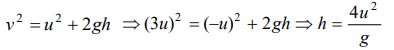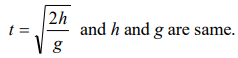## Motion in a Straight Line Questions and Answers Part-14

1. A ball P is dropped vertically and another ball Q is thrown horizontally with the same velocities from the same height and at the same time. If air resistance is neglected, then
a) Ball P reaches the ground first
b) Ball Q reaches the ground first
c) Both reach the ground at the same time
d) The respective masses of the two balls will decide the time

Explanation:2. A body is released from a great height and falls freely towards the earth. Another body is released from the same height exactly one second later. The separation between the two bodies, two seconds after the release of the second body is
a) 4.9m
b) 9.8m
c) 19.6 m
d) 24.5m

Explanation: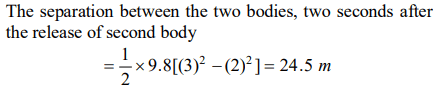3. An object is projected upwards with a velocity of 100 m/s. It will strike the ground after (approximately)
a) 10 sec
b) 20 sec
c) 15 sec
d) 5 sec

Explanation: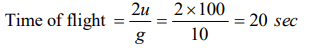4. A stone dropped from the top of the tower touches the ground in 4 sec. The height of the tower is about
a) 80 m
b) 40 m
c) 20 m
d) 160 m

Explanation:5. A body is released from the top of a tower of height h . It takes t sec to reach the ground. Where will be the ball after time $t\diagup 2$  sec
a) At $h\diagup 2$   from the ground
b) At $h\diagup4$   from the ground
c) Depends upon mass and volume of the body
d) At $3h\diagup 4$  from the ground

Explanation: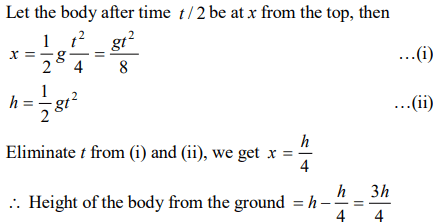6. A body is slipping from an inclined plane of height h and length l . If the angle of inclination is $\theta$ , the time taken by the body to come from the top to the bottom of this inclined plane is
a) $\sqrt{\frac{2h}{g}}$
b) $\sqrt{\frac{2l}{g}}$
c) $\frac{1}{\sin\theta}\sqrt{\frac{2h}{g}}$
d) $\sin\theta\sqrt{\frac{2h}{g}}$

Explanation: Force down the plane = mg sin $\theta$
Acceleration down the plane = g sin $\theta$7. A particle is projected up with an initial velocity of 80 ft/sec . The ball will be at a height of 96 ft from the ground after
a) 2.0 and 3.0 sec
b) Only at 3.0 sec
c) Only at 2.0 sec
d) After 1 and 2 sec

Explanation: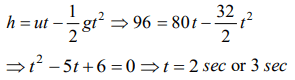8. A body falls from rest, its velocity at the end of first second is $\left(g=32 ft \diagup sec\right)$
a) 16 ft / sec
b) 32 ft / sec
c) 64 ft / sec
d) 24 ft / sec

Explanation: v = g * t = 32 * 1 = 32 ft /sec

9. A stone thrown upward with a speed u from the top of the tower reaches the ground with a velocity 3u . The height of the tower is
a) $3u^{2}\diagup g$
b) $4u^{2}\diagup g$
c) $6u^{2}\diagup g$
d) $9u^{2}\diagup g$